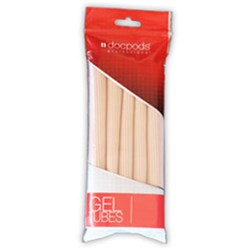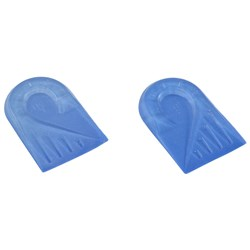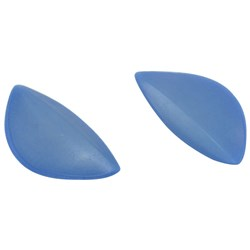# Orthotics & Footcare

Grid List
From
Increase value Decrease value
From
Increase value Decrease value
From
Increase value Decrease value
From
Increase value Decrease value
From
Increase value Decrease value
From
Increase value Decrease value
From
Increase value Decrease valueFrom
Increase value Decrease value
From
Increase value Decrease valueFrom
Increase value Decrease value
From
Increase value Decrease value
From
Increase value Decrease valueFrom
Increase value Decrease value
From
Increase value Decrease value
From
Increase value Decrease value
From
Increase value Decrease value
\$20.69
Increase value Decrease value
\$32.19
Increase value Decrease value
\$12.10
Increase value Decrease value
\$12.10
Increase value Decrease value
\$12.10
Increase value Decrease value
\$53.92
Increase value Decrease value
\$53.92
Increase value Decrease value
From
Increase value Decrease value
From
Increase value Decrease value
Grid List
##### Products to compare:
Comparing Products